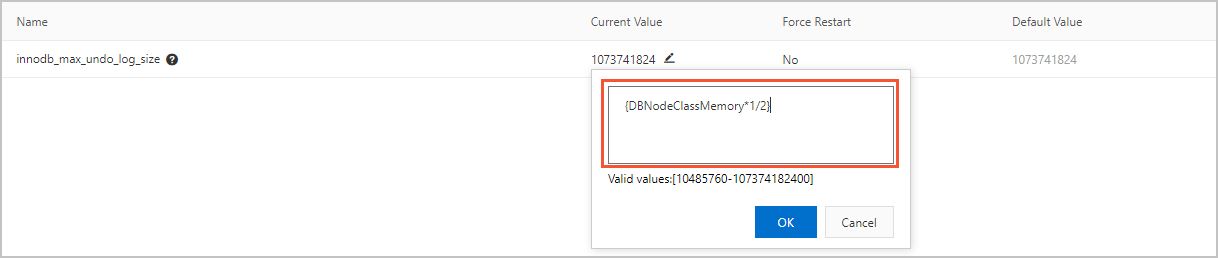PolarDB allows you to set the parameters of cluster specifications to expressions. Parameter values, which are expressions, dynamically change when specifications change. This ensures optimal performance and stability of a cluster.

## Usage notes

• Only numeric values can be specified in an expression. String values cannot be specified in an expression.

For example, if the valid values of the innodb_sort_buffer_size parameter are numeric values 65536 to 67108864, you can set this parameter to the following expression: `{DBNodeClassMemory*3/4}`. However, the valid values of the loose_recycle_bin parameter are ON and OFF, which are not numeric values. You cannot set this parameter to an expression.

Some parameters may have numeric values that do not carry numeric meaning, such as the valid values of the ssl parameter. The valid values of this parameter are 0, which specifies that SSL encryption is disabled, and 1, which specifies that SSL encryption is enabled. In this scenario, we recommend that you do not set this parameter to an expression.

• If the specifications of a cluster change, the value of a parameter that is set to an expression may become invalid. If the value becomes invalid, the final value of the parameter is based on the valid values.
For example, if `{DBNodeClassMemory*1/2}` is used to calculate the final value of the innodb_max_undo_log_size parameter and the final value of the parameter is within the range of valid values `10485760 to 107374182400 `, the final value is determined based on the following rules:
• If the memory of your cluster is upgraded to 256 GB or higher, the final value of `{DBNodeClassMemory*1/2}` is calculated by using the following formula: `256 GB × 1/2 = 128 GB = 137,438,953,472 bytes`. However, you cannot use this result as the final value because this result exceeds the specified range of valid values. Therefore, the final value of the innodb_max_undo_log_size parameter is 107374182400, which is the maximum valid value.
• If the memory of your cluster is lower than 256 GB, the final value of `{DBNodeClassMemory*1/2}` is within the range of valid values. Therefore, the final value of the innodb_max_undo_log_size parameter is the actual result of the calculation based on `{DBNodeClassMemory*1/2}`.
Note To ensure that the value of a parameter remains valid, we recommend that you check the valid values of the parameter before you set it to an expression, and consider how the value changes when specifications change.

## Supported expressions

The following table describes the expression syntax that is supported in PolarDB.

Category Remarks Format
Variables
• DBNodeClassIOPS: the input/output operations per second (IOPS) of a compute node. The value is an integer.
• DBNodeClassMemory: the memory size of a compute node. The value is an integer. Default unit: bytes.
• DBNodeClassCPU: the number of CPU cores of a compute node. The value is an integer.
• DBNodeClassConnections: the maximum number of connections supported by an instance. The value is an integer.
Note For more information about the compute node specifications, including the IOPS, memory size, number of CPU cores, and maximum number of connections, see Specifications of compute nodes.
`{DBNodeClassMemory*3/4}`: specifies that the value of the parameter must be equal to 75% of the memory size of the current compute node.
Operators
• Expression syntax: An expression is enclosed in a pair of braces (`{}`).
• Division operator (/): A dividend is divided by a divisor. The quotient is an integer. If the quotient is a decimal, only the whole number is used.
Syntax:
``dividend / divisor``
• Multiplication operator (*): A number multiplies another number. The product is an integer. If the product is a decimal, only the whole number is used.
Syntax:
``expression * expression``
Note The dividend, divisor, and multiplier must be integers. For example, `{DBNodeClassMemory*3/4}` is supported, but `{DBNodeClassMemory*0.75}` is not supported.
Functions
• The `GREATEST()` function returns the largest value from an array of integers or the largest value from an array of values calculated by an array of expressions.
Syntax:
``GREATEST(argument1, argument2,...argumentn)``
• The `LEAST()` function returns the smallest value from an array of integers or the smallest value from an array of values calculated by an array of expressions.
Syntax:
``LEAST(argument1, argument2,...argumentn)``
• The `SUM()` function adds an array of integers or the values calculated by an array of expressions.
Syntax:
``SUM(argument1, argument2,...argumentn)``
`LEAST({DBNodeClassMemory*1/2},10485760)`: specifies that the value of the parameter is the smaller value between 50% of the memory size of the current compute node and 10485760.

## How to use expressions

The procedure that is used to set parameters to expressions is the same as the procedure that is used to configure cluster parameters. For more information about the procedure, see Specify cluster parameters.

You need to enter only an expression when you modify Current Value. For example, you can set Current Value to `{DBNodeClassMemory*1/2}` for innodb_sort_buffer_size.